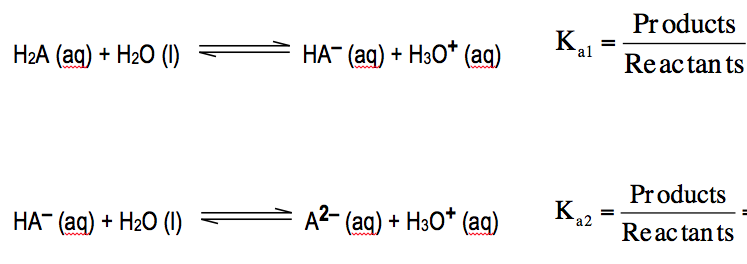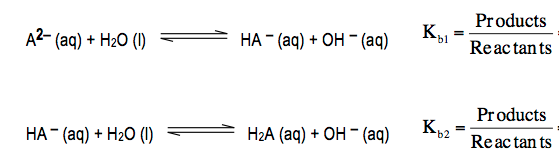Clutch Prep is now a part of Pearson
Ch.15 - Acid and Base EquilibriumWorksheetSee all chapters

# Diprotic Acid

See all sections
Sections
Identifying Acids and Bases
Arrhenius Acid and Base
Bronsted Lowry Acid and Base
Amphoteric
Lewis Acid and Base
pH and pOH
Ka and Kb
Ionic Salts
Diprotic Acid
Polyprotic Acid

A diprotic acid is an acid that can donate two hydronium ions (H+).

###### Diprotic Acids

Since a diprotic acid has two acidic hydrogens it would have 2 equilibrium equations.

Concept #1: Diprotic AcidsThe diprotic bases would also have 2 equilibrium equations.

Concept #2: Diprotic AcidsConcept #3: As a result of these equations for diprotic acids and bases the relationship between Ka and Kb can be established.

Example #1: Sulfurous acid, H2SO3, represents a diprotic acid with a Ka1 = 1.6 x 10-2 and Ka2 = 4.6 x 10-5. Calculate the pH and concentrations of H2SO3, HSO3 and SO32– when given 0.250 M H2SO3

Example #2: Determine the pH of 0.115 M Na2S. Hydrosulfuric acid, H2S, contains Ka1 = 1.0 x 10-7 and Ka2 = 9.1 x 10-8.